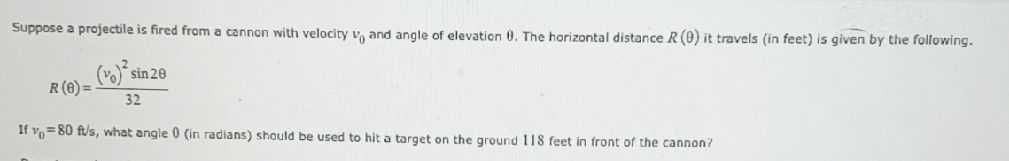### Still have math questions?

Trigonometry
QuestionSuppose a projectile is fired from a cannon with velocity $$v _ { 0 }$$ and angle of elevation $$\theta$$ . The horizontal distance $$R ( \theta )$$ it travels (in feet) is given by the following.

$$R ( \theta ) = \frac { ( v _ { 0 } ) ^ { 2 } \sin 2 \theta } { 32 }$$

If $$v _ { 0 } = 80 ft$$ /s, what angle $$0$$ (in radians) should be used to hit a target on the ground $$118$$ feet in front of the cannon?

$$R \theta = 118$$

$$V _ { 0 } = 80$$

$$118 = \frac { 80 ^ { 2 } \sin 2 \theta } { 32 }$$

$$0.59 = \sin 2 \theta$$

$$\frac { 1 } { 2 } \sin ^ { - 1 } ( 0 ^ { \circ } 59 ) = \theta$$

$$\theta = 0.316 rad$$

Solution
View full explanation on CameraMath App.